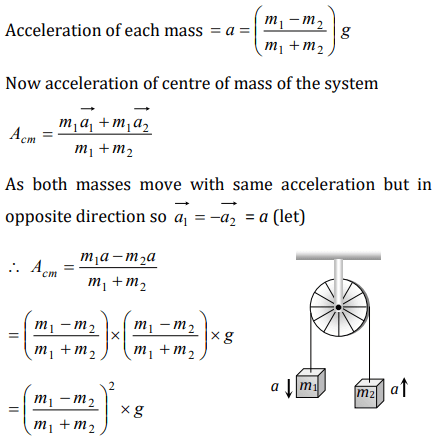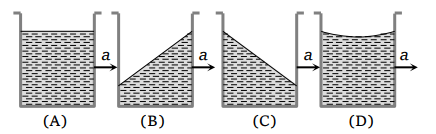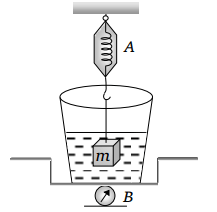## Laws of Motion Questions and Answers Part-20

1.Two masses M and M/2 are joint together by means of a light inextensible string passes over a frictionless pulley as shown in figure. When bigger mass is released the small one will ascend with an acceleration ofa) g/3
b) 3g/2
c) g/2
d) g

Explanation:2. Two masses $m_{1}$ and $m_{2}$ $\left(m_{1}>m_{2}\right)$   are connected by massless flexible and inextensible string passed over massless and frictionless pulley. The acceleration of centre of mass is
a) $\left(\frac{m_{1}-m_{2}}{m_{1}+m_{2}}\right)^{2}g$
b) $\frac{m_{1}-m_{2}}{m_{1}+m_{2}}g$
c) $\frac{m_{1}+m_{2}}{m_{1}-m_{2}}g$
d) Zero

Explanation:3. A vessel containing water is given a constant acceleration a towards the right, along a straight horizontal path. Which of the following diagram represents the surface of the liquida) A
b) B
c) C
d) D

Explanation: Due to acceleration in forward direction, vessel is an accelerated frame therefore a Pseudo force will be exerted in backward direction. Therefore water will be displaced in backward direction

4. A closed compartment containing gas is moving with some acceleration in horizontal direction. Neglect effect of gravity. Then the pressure in the compartment is
a) Same everywhere
b) Lower in front side
c) Lower in rear side
d) Lower in upper side

Explanation: The pressure on the rear side would be more due to fictitious force (acting in the opposite direction of acceleration) on the rear face. Consequently the pressure in the front side would be lowered.

5. A ship of mass $3\times 10^{7}kg$  initially at rest is pulled by a force of $5\times 10^{4}kg$  through a distance of 3 m. Assume that the resistance due to water is negligible, the speed of the ship is
a) 1.5 m/s
b) 60 m/s
c) 0.1 m/s
d) 5 m/s

Explanation:6. The mass of a body measured by a physical balance in a lift at rest is found to be m. If the lift is going up with an acceleration a, its mass will be measured as
a) $m\left(1-\frac{a}{g}\right)$
b) $m\left(1+\frac{a}{g}\right)$
c) m
d) Zero

Explanation: Mass measured by physical balance remains unaffected due to variation in acceleration due to gravity

7. Three weights W, 2W and 3W are connected to identical springs suspended from a rigid horizontal rod. The assembly of the rod and the weights fall freely. The positions of the weights from the rod are such that
a) 3W will be farthest
b) W will be farthest
c) All will be at the same distance
d) 2W will be farthest

Explanation: For W, 2W, 3W apparent weight will be zero because the system is falling freely. So the distances of the weight from the rod will be same

8. When forces $F_{1},F_{2},F_{3}$  are acting on a particle of mass m such that $F_{2}$  and $F_{3}$  are mutually perpendicular, then the particle remains stationary. If the force $F_{1}$ is now removed then the acceleration of the particle is
a) $F_{1}\diagup m$
b) $F_{2}F_{3}\diagup mF_{1}$
c) $\left(F_{2}-F_{3}\right)\diagup m$
d) $F_{2}\diagup m$

Explanation:9. The spring balance A reads 2 kg with a block m suspended from it. A balance B reads 5 kg when a beaker filled with liquid is put on the pan of the balance. The two balances are now so arranged that the hanging mass is inside the liquid as shown in figure. In this situationa) The balance A will read more than 2 kg
b) The balance B will read more than 5 kg
c) The balance A will read less than 2 kg and B will read more than 5 kg
d) Both b and c